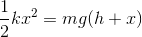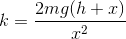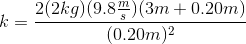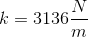# MCAT Physical : Other Forces

## Example Questions

### Example Question #424 : Mcat Physical Sciences

Two children are playing on an icy lake. Child 1 weighs 50kg, and child 2 weighs 38kg. Child 1 has a backpack that weighs 10kg, and child 2 has a backpack that weighs 5kg.

Over the course of the afternoon, they collide many times. Four collisions are described below.

Collision 1:

Child 1 starts from the top of a ramp, and after going down, reaches the lake surface while going 5m/s and subsequently slides into a stationary child 2. They remain linked together after the collision.

Collision 2:

Child 1 and child 2 are sliding in the same direction. Child 2, moving at 10m/s, slides into child 1, moving at 2m/s.

Collision 3:

The two children collide while traveling in opposite directions at 10m/s each.

Collision 4:

The two children push off from one another’s back, and begin moving in exactly opposite directions. Child 2 moves with a velocity of +8m/s.

Regarding the force of gravity pulling child 1 down the ramp, and the force of friction acting on the two children moving after the collision __________.

friction is a conservative force and gravity is a nonconservative force

friction is a nonconservative force and gravity is a conservative force

both are conservative forces

the conservative nature of forces depend on the bodies being acted upon

both are nonconservative forces

friction is a nonconservative force and gravity is a conservative force

Explanation:

Conservative forces are associated with a potential energy. The gravitational force that gives rise to the potential energy of child 1 at the top of the ramp is conserved, in a sense, as she converts it to kinetic energy during her trip down to the lake surface.

Friction has no associated potential energy, and so is considered nonconservative.

### Example Question #425 : Mcat Physical Sciences

Which of the following is not a conservative force?

Gravity

Electrostatic repulsion

Friction

Electrostatic attraction

Friction

Explanation:

Friction is a non-conservative force, meaning that the work it does depends on the path taken by the object. For example, moving a brick in a long zig-zag across the table will generate more heat from friction than moving it in a straight line across the table.

Electric and gravitational forces are conservative. This can be tested by knowing a constant equation to calculate the energy associated with these forces; such equations are applicable regardless of path. No such equation exists for frictional energy.

### Example Question #426 : Mcat Physical Sciences

A 2kg lead ball is loaded into a spring cannon and the cannon is set at a 45º angle on a platform. The spring has a spring constant of 100N/m and the ball and spring system is compressed by 1m before launch. While the ball is in flight air resistance can be neglected, and the ball finishes its flight by landing at a cushion placed some distance away from the cannon.

If air resistance could not be neglected, how should the positioning of the cushion change?

Closer

Further

Same Location

Not Enough Information to Determine

Closer

Explanation:

Air resistance is a form of friction that acts in the direction opposite motion, slowing down an object. If the ball spends less time in flight, it will travel less of a distance, requiring the cushion to be placed closer to the launch position.

### Example Question #427 : Mcat Physical Sciences

Which of the following fundamental forces is the weakest in magnitude?

Gravitational force

Weak force

Electromagnetic force

Nuclear force

Gravitational force

Explanation:

While one might expect the "weak" force to be the weakest of the four universal forces, the gravitational force is actually several orders of magnitude weaker than the weak force.

In order from strongest to weakest, the fundamental forces are the strong (nuclear) force, electromagnetic force, weak force, and gravitational force.

### Example Question #1 : Other Forces

Which of the following forces is not conservative?

Frictional

Gravitational

Elastic

Electrostatic

Frictional

Explanation:

Conservative forces are forces that do not lose energy to heat, sound, or light. Of these answers, energy is completely conserved and transferred from kinetic energy to potential energy, or vice versa. Gravitational forces, electrostatic forces, and elastic forces all work by providing a potential that will work in the same direction as the motion of an object or particle, allowing kinetic and potential energy to interconvert. Frictional forces lose energy as heat when sliding across a surface, and the more force (the more rough the surface), the more energy that is lost.

### Example Question #71 : Forces

A 2kg mass is suspended on a rope that wraps around a frictionless pulley attached to the ceiling with a mass of 0.01kg and a radius of 0.25m. The other end of the rope is attached to a massless suspended platform, upon which 0.5kg weights may be placed. While the system is initially at equilibrium, the rope is later cut above the weight, and the platform subsequently raised by pulling on the rope.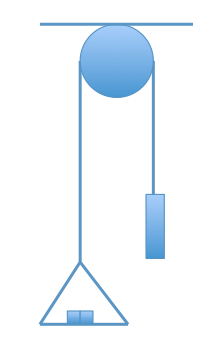If, instead of using one pulley to raise the platform after it falls, we decided to use two, how would the force required to lift the platform change?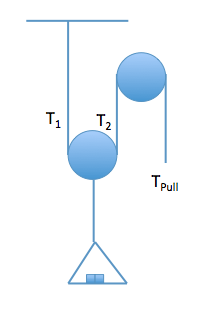Increase by 4 times

Decrease by 4 times

Decrease by 1/2

Increase by 1/2

Decrease by 1/2

Explanation:

One-half of the force will be required to pull the platform up in the new scenario. In the diagram below, now notice that the box has two upward tension forces due to the pulley attached directly to the box. These two tension forces act against the weight of the box, meaning that each tension force is one half of the weight of the box. Because tension is transmitted unchanged over the length of the rope, the tension a person would need to pull against would be half the weight of the box. This leads us to determine that half the force would be required to lift the box if two pulleys were used.### Example Question #72 : Forces

Two students (student X and student Y) lift a boulder vertically from point A to point B. Student X directly lifts the boulder from point A to point B, whereas student Y uses a pulley to lift the boulder. This allows student Y to apply a force (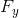) that is three times smaller than the force applied by student X (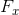). Both students apply force upwards and take the same amount of time to complete this task.

The vertical distance between point A and point B is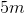.

Student Z uses a frictionless inclined plane to lift the boulder and has to apply only a third of. Which of the following is true regarding the inclined plane and the pulley (used by Student Y)?

The pulley has a greater mechanical advantage than the inclined plane

The inclined plane has a greater mechanical advantage than the pulley

The pulley and the inclined plane have the same mechanical advantage

The pulley and the inclined plane have the same mechanical advantage

Explanation:

The question states that: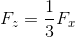Recall that the passage states thatis also one third of.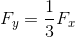The force exerted by student Y and student Z is the same.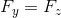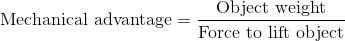The weight of the object is the same for both students because both of them are lifting the same boulder. The force applied is also the same; therefore, mechanical advantage for both machines is the same.

### Example Question #73 : Forces

Two students (student X and student Y) lift a boulder vertically from point A to point B. Student X directly lifts the boulder from point A to point B, whereas student Y uses a pulley to lift the boulder. This allows student Y to apply a force () that is three times smaller than the force applied by student X (). Both students apply force upwards and take the same amount of time to complete this task.

The vertical distance between point A and point B is.

If the pulley has a mechanical advantage ofand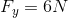, what is the weight of the boulder?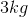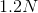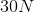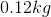Explanation:

The definition of mechanical advantage is:Rearranging this equation and solving for weight gives: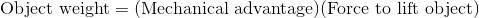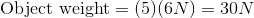Mechanical advantage is a unitless quantity. Remember the difference between weight and mass. Weight is a measure of force and has units of Newtons, whereas mass has units of kilograms.

### Example Question #74 : Forces

A 2kg object falls from a height of 3m onto a spring, which compresses 20cm. What is the spring constant?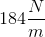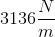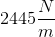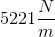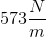Explanation:

Conservation of energy is the key here. Initial energy is all gravitational potential energy: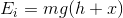Note that the final height change is equal to the height above the spring added to the displacement of the spring.

This is equal to the final energy, which is all spring potential energy: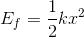Set these equations equal and solve for the spring constant.